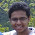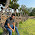Mar 6, 2015

Dividing Pizza with a Clock

Source: Alok Goyal Puzzle Page ( http://alokgoyal1971.com/ ) . Alok is ex-IIT Delhi, Partner at Helion VC

Problem:

Part I (Easy): Using a clock, divide a pizza among 12 people

Part II (Difficult): Using a clock, divide a pizza among 11 people?

1.a) use the 12 marks corresponding to 1'o clock, 2'o clock, ...., 12'o clock
b) use the 11 distinct and symmetric positions of the clock where the minute hand and hour hand overlap.

thought of a couple of further extensions:
c) (easy once previous parts are done) make 59 equal slices.
d) (mayb hard even after previous parts are done) make 43140 equal slices (not sure if the pizza would be edible by the time u finish this arduous task :D)

1.for c) i draw the analogy from part b, since hour and minute hand overlap 11 times, i think minute and second hand will overlap 59 times in an hour, so we can devide in 59 parts.

2.for c) i draw the analogy from part b, since hour and minute hand overlap 11 times, i think minute and second hand will overlap 59 times in an hour, so we can devide in 59 parts.

2.Omkar's b) was good
Another method ( not that easy though )
We will use the Hours hand to mark the cut and the minutes hand for keeping the count. The total 12 hours consists of 12 * 60 minutes = 720 minutes. If we divide the 720 minutes by 11 we get 65.45 If the hand is currently at 12'o' clock, we keep on extending the minutes hand by 65 minutes ( approx )

3.(d) was really Nice!
We make the count using minute hand and cut using hour hand. So we get 12*60 = 720min. But first cut can be made at start of each hour,so making it 719. Multiplying it with number of different second hand moves,we get 719*60 =43140 different slices.

4.Start when Hour, minute and second overlap on 12'O Clock. Then after 5 minute and 57 seconds, meaning when the minute hand is somewhat ahead of 1 and second hand is on 57 then take the angle b/w hour hand and minute hand. Which is approximately (360/11) degree.

Fraction Brainteaser

Source: Sent to me by Gaurav Sinha Problem: Siddhant writes a Maths test and correctly answers 5 out of 6 Arithmetic questions and 20...